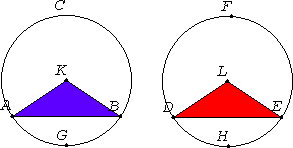Proposition 29

In equal circles straight lines that cut off equal circumferences are equal.

Let ABC and DEF be equal circles, and in them let equal circumferences BGC and EHF be cut off. Join the straight lines BC and EF.I say that BC equals EF.

III.1

Take the centers K and L of the circles. Join BK, KC, EL, and LF.

III.27

Now, since the circumference BGC equals the circumference EHF, the angle BKC also equals the angle ELF.

I.4

And, since the circles ABC and DEF are equal, the radii are also equal, therefore the two sides BK and KC equal the two sides EL and LF, and they contain equal angles, therefore the base BC equals the base EF.

Therefore in equal circles straight lines that cut off equal circumferences are equal.

Q.E.D.

Guide

This proposition is used in IV.11 and IV.15.